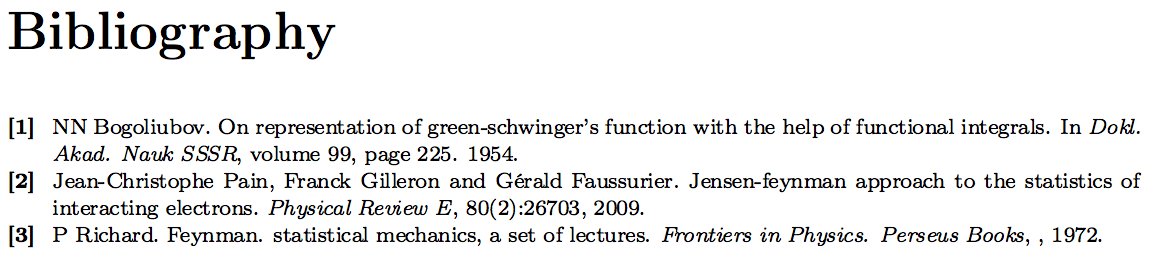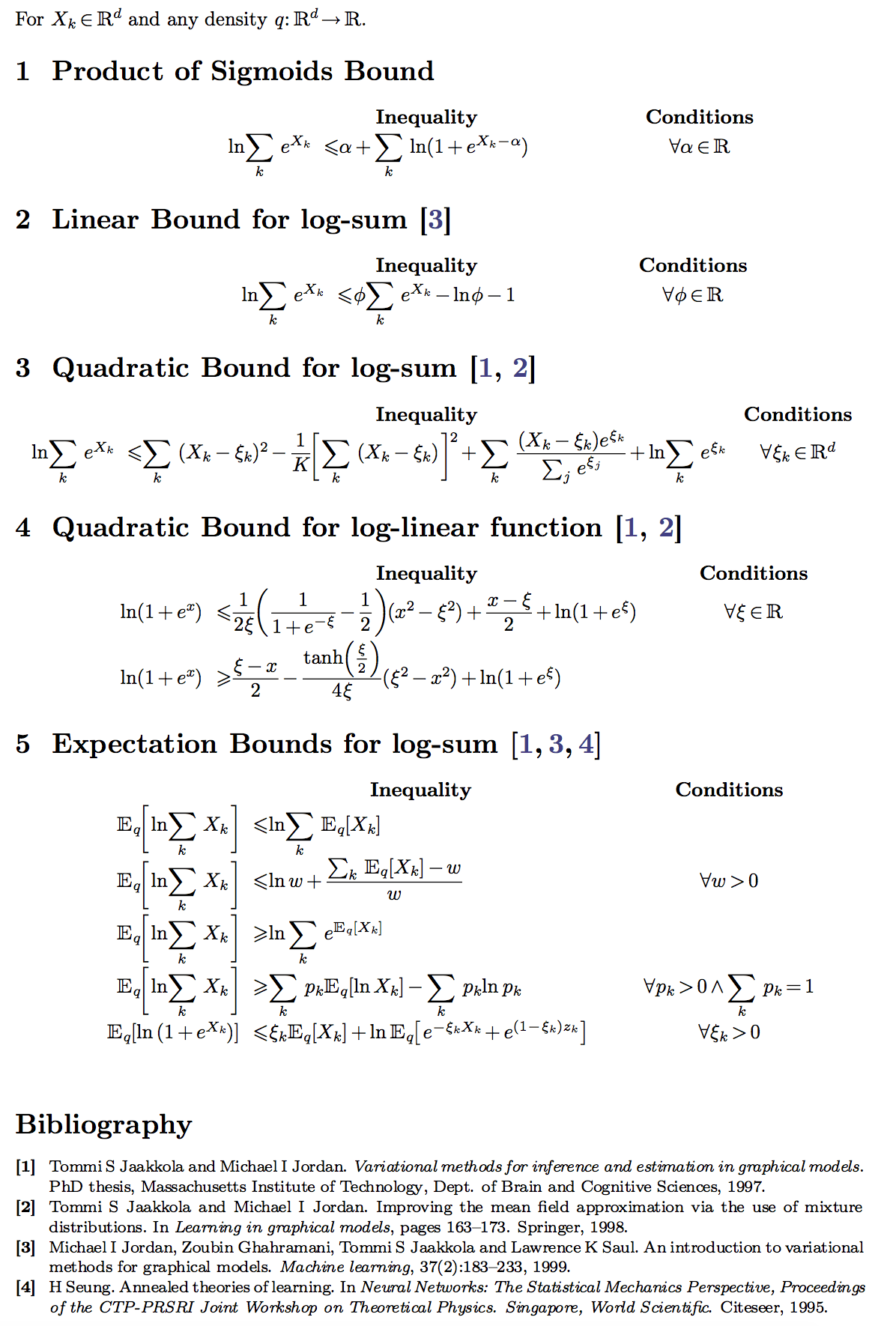# Partition Functions and Higher-order Jensen Inequalities (3/3)

When trying to compute variational bounds (as derived in the previous post), a naive attempt to approximate the involved expectations (e.g. using a Taylor expansion) may destroy the bound.

This is where the Higher-order Jensen-Feynman inequality comes in. It allows us to do a higher-order polynomial expansion without destroying the variational bound.# Partition Functions and the Jensen-Feynman Inequalities (2/3)# Useful Inequalities for Variational Inference

Variational Inference is a technique which consists in bounding the log-likelihood ln p(x) defined by a model with latent variables p(x,z)=p(x|z)p(z) through the introduction of  a variational distribution q(z|x) with same support as p(z):Often the expectations in the bound F(x) (aka, ELBO or Free Energy) cannot be solved analytically.

In some cases, we can make use of a few handful inequalities which I quickly summarize below.

Some of these inequalities introduce new variational parameters. Those should be optimized jointly with all the other parameters to minimize the ELBO.